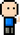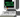# Josh Meets Computer# Chi-squared test for Feature Selection

October 8, 2018

## Determining Feature Importance

Are there certain features that play a larger role in the final prediction? Chi-squared test is one approach for better understanding the importance of each feature by giving you an idea of how much impact each feature will have on the final prediction

## What Is It?

``````Chi-squared is used in a "Goodness of fit" test that tells you if your sample data fits within the distribution of a given population. Does your data represent what you would expect to find from this given population?
Chi-squared is also used to test for independence -- comparing two variables in a table to see if they are related to each other. Do the distributions of these variables differ from each other? A small chi square test statistic means there is a relationship (the observed data fits the latter very well). A large chi square statistic means there was a poor fit, and therefore not much of a relationship``````

## How to calculate:

``````import pandas as pd
import numpy as np
def chi_squared_test(dataframe, observed, expected):
'''
Function recieves in a dataframe that contains a
column that represents your oberserved value, and
a column that represents your expected value. It
then calculates and returns the chi-squared value.

args:
dataframe <pandas> : Pandas Dataframe
observed <string> : column name of your observed value
expected <string> : column name of your expected value

returns:
chi-square <float> : chi squared value

Notes:
If the chi-squared value is about zero, then there is a
near perfect relationship between the two. Take this chi
squared value and compare it to a critical value form a
chi-squared table.
'''

diff = dataframe[observed] - dataframe[expected]
dfff = np.power(diff, 2)
diff = np.divide(diff, dataframe[expected])

chi_squared = np.sum(diff, axis=1).squeeze()

return chi_squared``````

## Applications

``Chi-squared helsp show the relationship between two categorical variables. ``